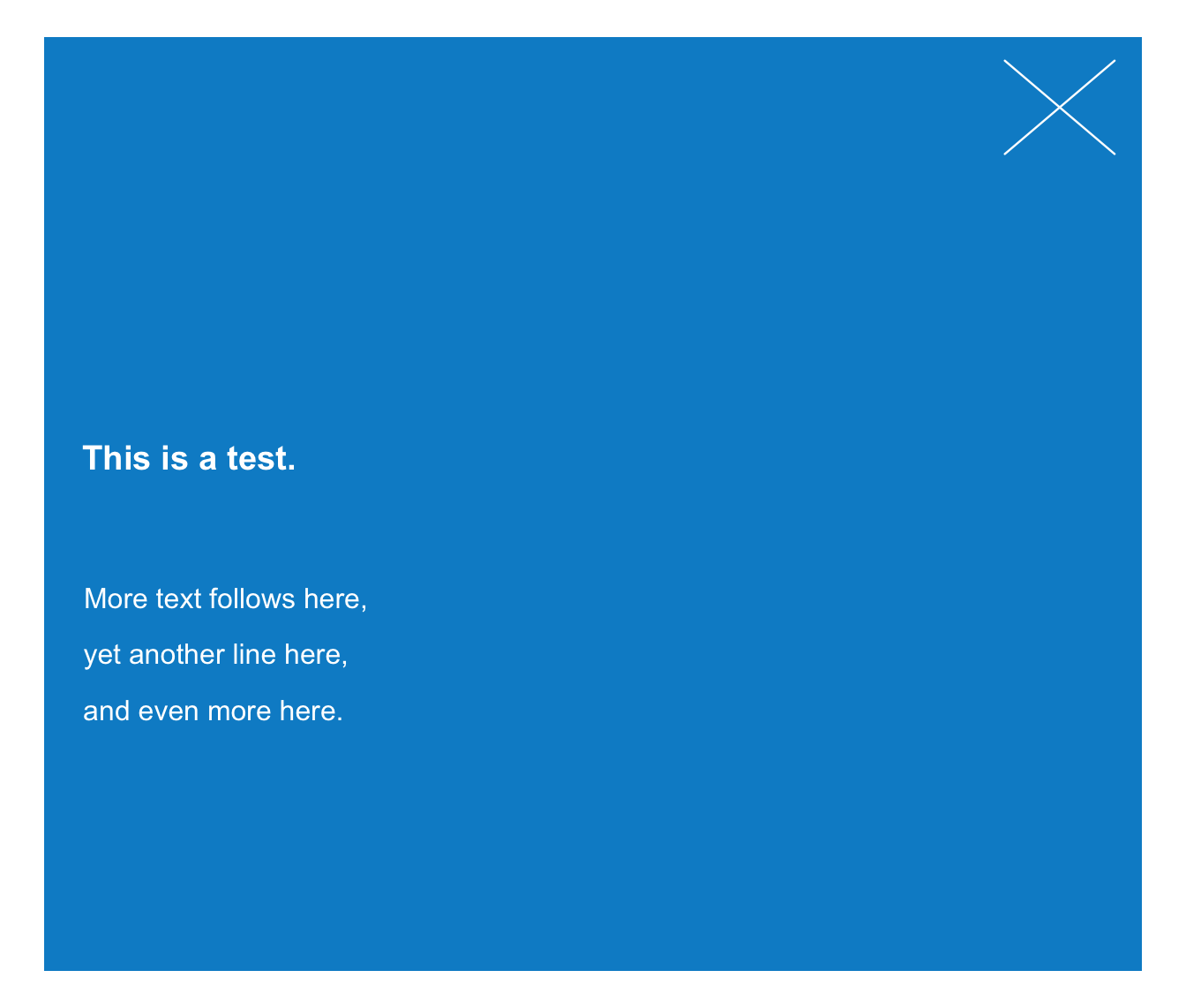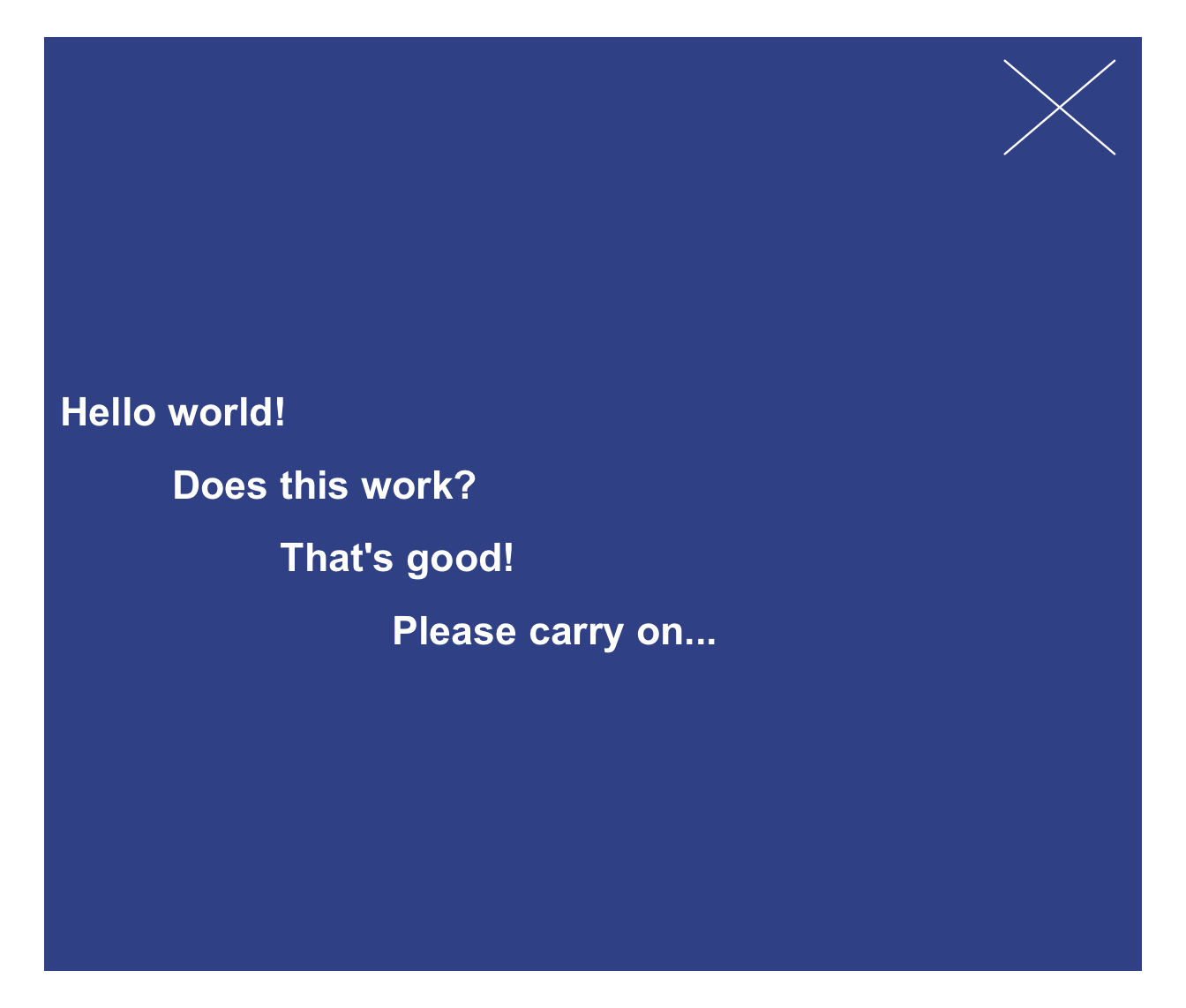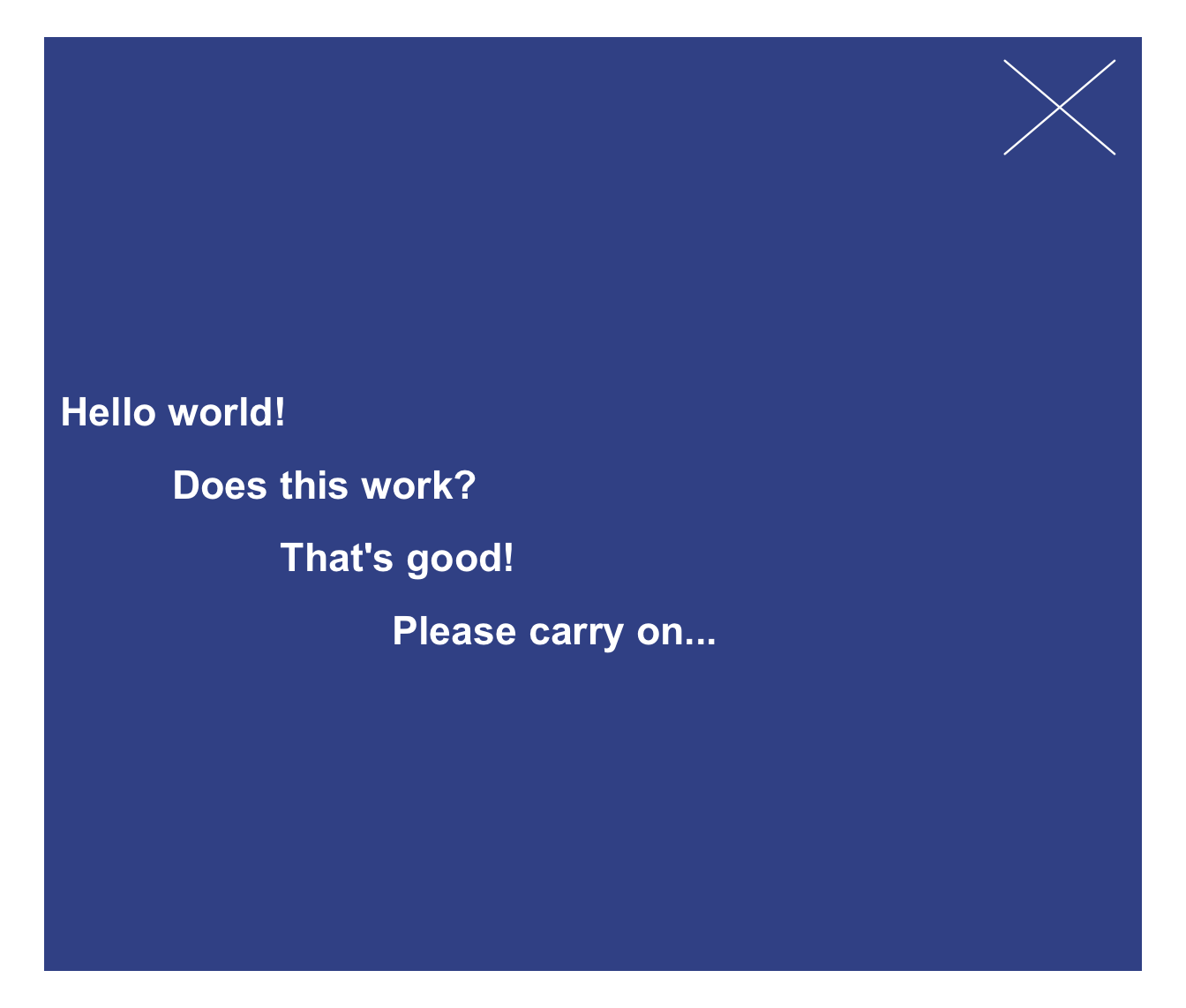post plots 1 or more text strings (provided as a character vector labels) to an (existing or new) xbox.

post(
labels,
x = 0.03,
y = 0.55,
x_layout = NA,
y_layout = "even",
col = "white",
col_bg = Seeblau,
cex = 1,
font = 1,
new_plot = "none"
)

## Arguments

labels A character vector specifying the text labels to be plotted. A numeric vector of x-coordinates at which the text labels in labels should be written. If the lengths of x and y differ, the shorter one is recycled. Default: x = .03. A numeric vector of y-coordinates at which the text labels in labels should be written. If the lengths of x and y differ, the shorter one is recycled. Default: y = .55. An optional numeric vector or character string to control the horizontal positions of labels. Numeric values are interpreted as increments to values of x and recycled (to enable stepwise or alternating patterns). 3 character string options are: "center" (i.e., center wrt. first label or plot center), "left" (i.e., left wrt. first label or plot center), "right" (i.e., right wrt. first label or plot center). Default: x_layout = NA (i.e., using values of x). A numeric value or character string to control the vertical positions of labels. Numeric values are interpreted as increments to values of y and recycled (to enable stepwise or alternating patterns). 2 character string options are: "even" (i.e., even distribution of labels across available y-space) and "flush" (i.e., no space between adjacent labels, i.e., y_layout = 0). Default: y_layout = "even". The color(s) of the text label(s). Default: col_lbl = "white". The background color(s) of the xbox. Default: col_bg = Seeblau. Numeric character expansion factor(s), multiplied by par("cex") to yield the character size(s). Default: cex = 1.0. The font type(s) to be used. Default: font = 1 (i.e., plain text). Should a new plot be generated? Set to "xbox" to plot to a basic xbox (with square dimensions, i.e., dim = c(1, 1)). Default: new_plot = "none" (i.e., assumes a pre-existing xbox).

## Details

The positions of the text elements in labels can be specified by providing their coordinates (as x and y arguments) or by providing an initial position and an y_layout (see below).

Text formatting parameters (like col, col_bg, cex, font) are recycled to match length(labels).

post uses the base graphics system graphics::.

xbox to create a new xbox (without text).

Other text functions: mark(), uline(), url_unikn()

## Examples

post(labels = "Post this line with default settings.", new_plot = "xbox")# Create a new xbox:
post(labels = "This is a test.", new_plot = "xbox",
cex = 1.2, font = 2, col_bg = pal_seeblau[])

# Add text to an existing xbox:
post(labels = c("More text follows here,",
"yet another line here,",
"and even more here."),
y = .4, y_layout = .04,
new_plot = "none")# Using x_layout and y_layout:
post(labels = c("Ene,", "mene, miste,", "es rappelt", "in der Kiste."),
cex = 1.4, font = 2, col = "white", col_bg = Pinky,
x = .1, y = .5, x_layout = "left", y_layout = .05, new_plot = "xbox")post(labels = c("Hello world!", "Does this work?", "That's good!", "Please carry on..."),
cex = 1.4, font = 2, col = "white", col_bg = Karpfenblau,
x = .01, y = .6, x_layout = .10, y_layout = .05, new_plot = "xbox")